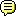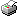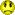Register (or login) on our website and you will not see this ad.cheshire_man(knowledge is power) Sat 20-Apr-13 22:45:49# Excel function CountIfS returning incorrect result

I have an Excel spreadsheet where I wish to count the number of cells in each of 12 columns containing the value 5. All the cells in the relevant columns contain a number from 1 to 5 or are blank. They are all formatted as General.

So I have the formula
=COUNTIFS(G3:G39,5,L3:L39,5,Q3:Q39,5,V3:V39,5,AA3:AA39,5,AF3:AF39,5,AK3:AK39,5,AP3:AP39,5,AU3:AU39,5,AZ3:AZ39,5,BE3:BE39,5,BJ3:BJ39,5)
It doesn't (seem to) make any difference whether the criteria is expressed as 5 or "5" or "=5".

4 of the columns contain an occurrence of the tested value, but the formula returns 0

If I use the formula
=COUNTIFS(L3:L39,5,Q3:Q39,5)
which evaluates 2 of the columns actually containing the value 5 it returns 1. And, I'm sure this is a clue but I'm darned if I can see it, if I delete either of the 5 values the result is 0. So it's clearly testing both columns.

When editing the formula Excel correctly highlights all 12 columns.

Any thoughts?

Edit:

I've just tried changing the formula to summing the results of 12 separate CountIf functions
=COUNTIF(G3:G39,5)+COUNTIF(L3:L39,5)+COUNTIF(Q3:Q39,5)+COUNTIF(V3:V39,5)+COUNTIF(AA3:AA39,5)+COUNTIF(AF3:AF39,5)+COUNTIF(AK3:AK39,5)+COUNTIF(AP3:AP39,5)+COUNTIF(AU3:AU39,5)+COUNTIF(AZ3:AZ39,5)+COUNTIF(BE3:BE39,5)+COUNTIF(BJ3:BJ39,5)
and it correctly gives the result 4.

So have I misunderstood the CountIfS function?

Tony
We have more and more laws, and less and less enforcement

Edited by cheshire_man (Sat 20-Apr-13 22:54:08)XRaySpeX(eat-sleep-adslguide) Sun 21-Apr-13 00:07:36## Re: Excel function CountIfS returning incorrect result

Sorry, don't have this plural COUNTIFS in my Excel. Use the singular COUNTIF; you know it works.

What is the syntax/definition of COUNTIFS?

1999: Freeserve 48K Dial-Up => 2005: Wanadoo 1 Meg BB => 2007: Orange 2 Meg BB => 2008: Orange 8 Meg LLU => 2010: Orange 16 Meg LLU => 2011: Orange 20 Meg WBC## Re: Excel function CountIfS returning incorrect result

It seems COUNTIFS is basically an 'AND' function, in that it counts the number of occurrences of a value in the first range, then counts the number of occurrences of another values (or the same value) in the next range, and so on. It stops if it hits a null result for any given range, and reports a zero.

Think of it as "How often do all the conditions match?"

~ Camieabz ~

All Connection Data ~ Some plusnet links

Register (or login) on our website and you will not see this ad.## Re: Excel function CountIfS returning incorrect result

Here's an example of how it might be used.

Obviously, the same result can be achieved by summing all the rows or columns then using COUNTIF to see how often if the desired value exists. It looks like Harry and Paul's jackets are on shoogly pegs.~ Camieabz ~

All Connection Data ~ Some plusnet linkscheshire_man(knowledge is power) Sun 21-Apr-13 14:49:13## Re: Excel function CountIfS returning incorrect result

Sorry, it's in Excel 2007 on AFAIK.

Tony
We have more and more laws, and less and less enforcementcheshire_man(knowledge is power) Sun 21-Apr-13 14:49:50## Re: Excel function CountIfS returning incorrect result

In reply to a post by camieabz:
It seems COUNTIFS is basically an 'AND' function, in that it counts the number of occurrences of a value in the first range, then counts the number of occurrences of another values (or the same value) in the next range, and so on. It stops if it hits a null result for any given range, and reports a zero.

Think of it as "How often do all the conditions match?"
That makes a lot of sense. Thank you sir.

Tony
We have more and more laws, and less and less enforcement